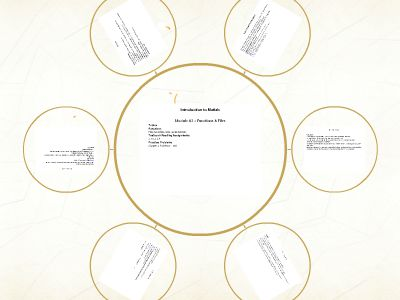Publications: 30 | Followers: 0

## EE261 Lecture Notes (electronic) - Montana State University

Publish on Category: Birds 0

Introduction to Matlab
Module #3 – Functions & FilesTopicsFunctionsFiles (variables, data, spreadsheets)Textbook Reading Assignments3.1-3.2, 3.4Practice ProblemsChapter 3 Problems: tbd
1) Functions
Functions- Matlab gives you the ability to create your own user-defined functions.- A function is a file that exists that performs a specific task- It is different from a script in that it returns the results of a computation andthe result can be assigned to variables.- Variables used within the function file are not seen by the rest of the program (i.e., they don’t showup in the workspace) so functions can be called without altering existing session variables.- You name the file the same name as the function and give it a *.m extension
1) Functions
Syntaxfunction [output variables] = function_name(input variables)- “function” is a keyword and must be placed on the first line of the file- the square brackets represent what variables in the function file will be returned at the function call- “function_name” is user-defined. Your *.m file should have the same name- the parenthesis hold the inputs that will be passed to the function.
1) Functions
Example Function (1 output)- let’s make a function that will evaluate this expression:y = x2+ 5- the userwilpass in the value ofxand the function will returny.- let’s call the function “eq_test.m”function[y] =eq_test(x)y = x^2 +5;- this can be called using: >>eq_test(1) or>> c =eq_test(2)
1) Functions
Example Function (2 outputs)- let’s make a function that will calculate the area and circumference of a circle.function[A, C] =circle_comp(r)A = pi*r.^2;C = 2*pi*r;- when calling this, we need to provide two variables for A and C to be assigned to.>> [Area, Circum] =circle_comp(3)- if two variables are not provided, only the first output will be returned>>circle_comp(2)ans= 12.5
2) Files
Importing ASCII Files- You can load in an ASCII file using the command>> load filename.dat- A matrix will be created with the rows and columns found in this file with the namefilename- The file is assumed to be space delimited and contain to headers- TheImport Wizardallows more complex data files to be read in (i.e., headers, different delimiters…)Importing Spreadsheet Files- You can import from excel (*.xls) using the command:>>xlsread(‘filename’)
Lab 3 Exercise Problems
For each of these exercises, you will create a script file. Your script file will perform the calculations and then display the answers to the workspace.Create a directory on your Z drive called “Z:\Matlab_Course\Lab03”Change yourpwdto “Z:\Matlab_Course\Lab03”(>>cdZ:\Matlab_Course\Lab03)Perform the following exercises:ASCII Import- download the ASCII file from the course website into your working directory- import the data and plot it.XLS Import- download the XLS spreadsheet file from the course website into your working directory- import the data and plot it.3.9- Create a script file called Lab03_3d9.m to perform the computations3.10- Create a script file called Lab03_3d10.m to perform the computations

0

Embed

Share